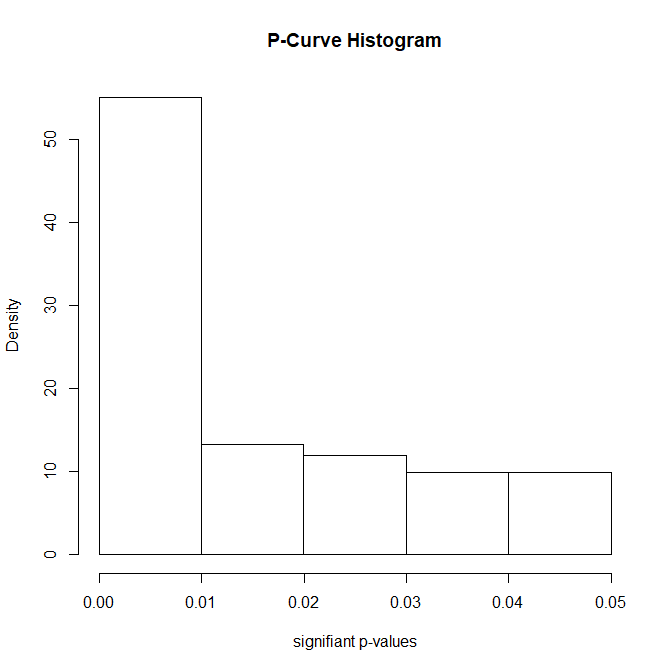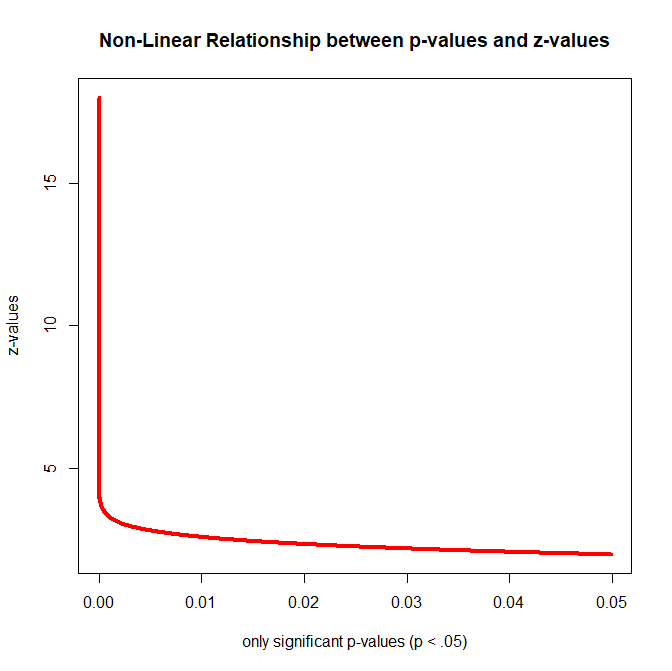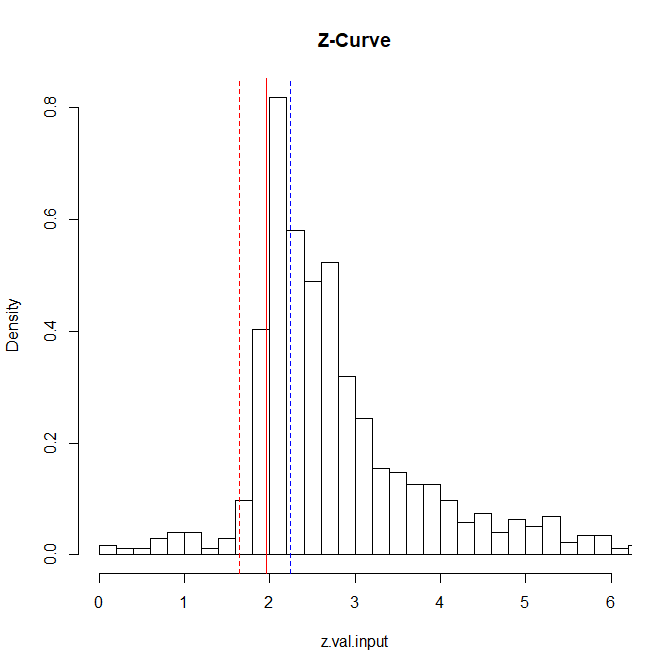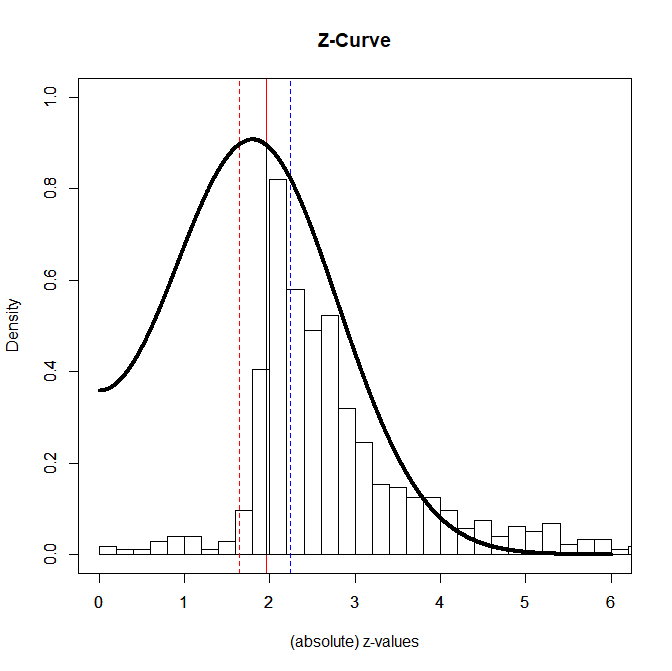# Visual Inspection of Strength of Evidence: P-Curve vs. Z-Curve

Statistics courses often introduce students to a bewildering range of statistical test.  They rarely point out how test statistics are related.  For example, although t-tests may be easier to understand than F-tests, every t-test could be performed as an F-test and the F-value in the F-test is simply the square of the t-value (t^2 or t*t).

At an even more conceptual level, all test statistics are ratios of the effect size (ES) and the amount of sampling error (ES).   The ratio is sometimes called the signal (ES) to noise (ES) ratio.  The higher the signal to noise ratio (ES/SE), the stronger the observed results deviate from the hypothesis that the effect size is zero.  This hypothesis is often called the null-hypothesis, but this terminology has created some confusing.  It is also sometimes called the nil-hypothesis the zero-effect hypothesis or the no-effect hypothesis.  Most important, the test-statistic is expected to average zero if the same experiment could be replicated a gazillion times.

The test statistics of statistical tests cannot be directly compared.  A t-value of 2 in a study with N = 10 participants provides weaker evidence against the null-hypothesis than a z-score of 1.96.  and an F-value of 4 with df(1,40) provides weaker evidence than an F(10,200) = 4 result.  It is only possible to compare test values directly that have the same sampling distribution (z with z, F(1,40) with F(1,40), etc.).

There are three solutions to this problem. One solution is to use effect sizes as the unit of analysis. This is useful if the aim is effect size estimation.  Effect size estimation has become the dominant approach in meta-analysis.  This blog post is not about effect size estimation.  I just mention it because many readers may be familiar with effect size meta-analysis, but not familiar with meta-analysis of test statistics that reflect the ratio of effect size and sampling error (Effect size meta-analysis: unit = ES; Test Statistic Meta-Analysis: unit ES/SE).

P-Curve

There are two approaches to standardize test statistics so that they have a common unit of measurement.  The first approach goes back to Ronald Fisher, who is considered the founder of modern statistics for researchers.  Following Fisher it is common practice to convert test-statistics into p-values (for this blog post assumes that you are familiar with p-values).   P-values have the same meaning independent of the test statistic that was used to compute them.   That is, p = .05 based on a z-test, t-test, or an F-test provide equally strong evidence against the null-hypothesis (Bayesians disagree, but that is a different story).   The use of p-values as a common metric to examine strength of evidence (evidential value) was largely forgotten, until Simonsohn, Simmons, and Nelson (SSN) used p-values to develop a statistical tool that takes publication bias and questionable research practices into account.  This statistical approach is called p-curve.  P-curve is a family of statistical methods.  This post is about the p-curve plot.

A p-curve plot is essentially a histogram of p-values with two characteristics. First, it only shows significant p-values (p < .05, two-tailed).  Second, it plots the p-values between 0 and .05 with 5 bars.  The Figure shows a p-curve for Motyl et al.’s (2017) focal hypothesis tests in social psychology.  I only selected t-test and F-tests from studies with between-subject manipulations.The main purpose of a p-curve plot is to examine whether the distribution of p-values is uniform (all bars have the same height).  It is evident that the distribution for Motyl et al.’s data is not uniform.  Most of the p-values fall into the lowest range between 0 and .01. This pattern is called “rigth-skewed.”  A right-skewed plot shows that the set of studies has evidential value. That is, some test statistics are based on non-zero effect sizes.  The taller the bar on the left is, the greater the proportion of studies with an effect.  Importantly, meta-analyses of p-values do not provide information about effect sizes because p-values take effect size and sampling error into account.

The main inference that can be drawn from a visual inspection of a p-curve plot is how unlikely it is that all significant results are false positives; that is, the p-value is below .05 (statistically significant), but this strong deviation from 0 was entirely due to sampling error, while the true effect size is 0.

The next Figure also shows a plot of p-values.  The difference is that it shows the full range of p-values and that it differentiates more between p-values because p = .09 provides weaker evidence than p = .0009.The histogram shows that most p-values are below p < .001.  It also shows very few non-significant results.  However, this plot is not more informative than the actual p-curve plot. The only conclusion that is readily visible is that the distribution is not uniform.

The main problem with p-value plots is that p-values do not have interval scale properties.  This means, the difference between p = .4 and p = .3 is not the same as the difference between p = .10 and p = .00 (e.g., .001).

Z-Curve

Stouffer developed an alternative method to Fisher’s p-value meta-analysis.  Every p-value can be transformed into a z-scores that corresponds to a particular p-value.  It is important to distinguish between one-sided and two-sided p-values.  The transformation requires the use of one-sided p-values, which can be obtained by simply dividing a two-sided p-value by 2.  A z-score of -1.96 corresponds to a one-sided p-value of 0.025 and a z-score of 1.96 corresponds to a one-sided p-values of 0.025.  In a two sided test, the sign no longer matters and the two p-values are added to yield 0.025 + 0.025 = 0.05.

In a standard meta-analysis, we would want to use one-sided p-values to maintain information about the sign.  However, if the set of studies examines different hypothesis (as in Motyl et al.’s analysis of social psychology in general) the sign is no longer important.   So, the transformed two-sided p-values produce absolute (only positive) z-scores.

The formula in R is Z = -qnorm(p/2)   [p = two.sided p-value]

For very strong evidence this formula creates problems. that can be solved by using the log.P=TRUE option in R.

Z = -qnorm(log(p/2), log.p=TRUE)The plot shows the relationship between z-scores and p-values.  While z-scores are relatively insensitive to variation in p-values from .05 to 1, p-values are relatively insensitive to variation in z-scores from 2 to 15.The next figure shows the relationship only for significant p-values.  Limiting the distribution of p-values does not change the fact that p-values and z-values have very different distributions and a non-linear relationship.

The advantage of using (absolute) z-scores is that z-scores have ratio scale properties.  A z-score of zero has real meaning and corresponds to the absence of evidence for an effect; the observed effect size is 0.  A z-score of 2 is twice as strong as a z-score of 1. For example, given the same sampling error the effect size for a z-score of 2 is twice as large as the effect size for a z-score of 1 (e.g., d = .2, se = .2, z = d/se = 1,  d = 4, se = .2, d/se = 2).

It is possible to create the typical p-curve plot with z-scores by selecting only z-scores above z = 1.96. However, this graph is not informative because the null-hypothesis does not predict a uniform distribution of z-scores.   For z-values the central tendency of z-values is more important.  When the null-hypothesis is true, p-values have a uniform distribution and we would expect an equal number of p-values between 0 and 0.025 and between 0.025 and 0.050.   A two-sided p-value of .025 corresponds to a one-sided p-value of 0.0125 and the corresponding z-value is 2.24

p = .025
-qnorm(log(p/2),log.p=TRUE)
 2.241403

Thus, the analog to a p-value plot is to examine how many significant z-scores fall into the region from 1.96 to 2.24 versus the region with z-values greater than 2.24.The histogram of z-values is called z-curve.  The plot shows that most z-values are in the range between 1 and 6, but the histogram stretches out to 20 because a few studies had very high z-values.  The red line shows z = 1.96. All values on the left are not significant with alpha = .05 and all values on the right are significant (p < .05).  The dotted blue line corresponds to p = .025 (two tailed).  Clearly there are more z-scores above 2.24 than between 1.96 and 2.24.  Thus, a z-curve plot provides the same information as a p-curve plot.  The distribution of z-scores suggests that some significant results reflect true effects.

However, a z-curve plot provides a lot of additional information.  The next plot removes the long tail of rare results with extreme evidence and limits the plot to z-scores in the range between 0 and 6.  A z-score of six implies a signal to noise ratio of 6:1 and corresponds to a p-value of p = 0.000000002 or 1 out of 2,027,189,384 (~ 2 billion) events. Even particle physics settle for z = 5 to decide that an effect was observed if it is so unlikely for a test result to occur by chance.

> pnorm(-6)*2
 1.973175e-09

Another addition to the plot is to include a line that identifies z-scores between 1.65 and 1.96.  These z-scores correspond to two-sided p-values between .05 and .10. These values are often published as weak but sufficient evidence to support the inference that a (predicted) effect was detected. These z-scores also correspond to p-values below .05 in one-sided tests.A major advantage of z-scores over p-values is that p-values are conditional probabilities based on the assumption that the null-hypothesis is true, but this hypothesis can be safely rejected with these data.  So, the actual p-values are not important because they are conditional on a hypothesis that we know to be false.   It is like saying, I would be a giant if everybody else were 1 foot tall (like Gulliver in Lilliput), but everybody else is not 1 foot tall and I am not a giant.

Z-scores are not conditioned on any hypothesis. They simply show the ratio of the observed effect size and sampling error.  Moreover, the distribution of z-scores tell us something about the ratio of the true effect sizes and sampling error.  The reason is that sampling error is random and like any random variable has a mean of zero.  Therefore, the mode, median, or mean of a z-curve plot tells us something about ratio of the true effect sizes and sampling error.  The more the center of a distribution is shifted to the right, the stronger is the evidence against the null-hypothesis.  In a p-curve plot, this is reflected in the height of the bar with p-values below .01 (z > 2.58), but a z-curve plot shows the actual distribution of the strength of evidence and makes it possible to see where the center of a distribution is (without more rigorous statistical analyses of the data).

For example, in the plot above it is not difficult to see the mode (peak) of the distribution.  The most common z-values are between 2 and 2.2, which corresponds to p-values of .046 (pnorm(-2.2)*2) and .028 (pnorm(-2.2)*2).   This suggests that the modal study has a ratio of 2:1 for effect size over sampling error.

The distribution of z-values does not look like a normal distribution. One explanation for this is that studies vary in sampling errors and population effect sizes.  Another explanation is that the set of studies is not a representative sample of all studies that were conducted.   It is possible to test this prediction by trying to fit a simple model to the data that assumes representative sampling of studies (no selection bias or p-hacking) and that assumes that all studies have the same ratio of population effect size over sampling error.   The median z-score provides an estimate of the center of the sampling distribution.  The median for these data is z = 2.56.   The next picture shows the predicted sampling distribution of this model, which is an approximately normal distribution with a folded tail.A comparison of the observed and predicted distribution of z-values shows some discrepancies. Most important is that there are too few non-significant results.  This observation provides evidence that the results are not a representative sample of studies.  Either non-significant results were not reported or questionable research practices were used to produce significant results by increasing the type-I error rate without reporting this (e.g., multiple testing of several DVs, or repeated checking for significance during the course of a study).

It is important to see the difference between the philosophies of p-curve and z-curve. p-curve assumes that non-significant results provide no credible evidence and discards these results if they are reported.  Z-curve first checks whether non-significant results are missing.  In this way, p-curve is not a suitable tool for assessing publication bias or other problems, whereas even a simple visual inspection of z-curve plots provides information about publication bias and questionable research practices.The next graph shows a model that selects for significance.  It no longer attempts to match the distribution of non-significant results.  The objective is only to match the distribution of significant z-values.  You can do this by hand and simply try out different values for the center of the normal distribution.  The lower the center, the more z-scores are missing because they are not significant.  As a result, the density of the predicted curve needs to be adjusted to reflect the fact that some of the area is missing.

center.z = 1.8  #pick a value
z = seq(0,6,.001)  #create the range of z-values
y = dnorm(z,center.z,1) + dnorm(z,-center.z,1)  # get the density for a folded normal
y2 = y #duplicate densities
y2[x < 1.96] = 0   # simulate selection bias, density for non-significant results is zero
scale = sum(y2)/sum(y)  # get the scaling factor so that area under the curve of only significant results is 1.
y = y / scale   # adjust the densities accordingly

# draw a histogram of z-values
# input is  z.val.input
# example; z.val.input = rnorm(1000,2)
hist(z.val.input,freq=FALSE,xlim=c(0,6),ylim=c(0,1),breaks=seq(0,20,.2), xlab=””,ylab=”Density”,main=”Z-Curve”)

abline(v=1.96,col=”red”)   # draw the line for alpha = .05 (two-tailed)
abline(v=1.65,col=”red”,lty=2)  # draw marginal significance (alpha = .10 (two-tailed)

par(new=TRUE) #command to superimpose next plot on histogram

# draw the predicted sampling distribution
plot(x,y,type=”l”,lwd=4,ylim=c(0,1),xlim=c(0,6),xlab=”(absolute) z-values”,ylab=””)

Although this model fits the data better than the previous model without selection bias, it still has problems fitting the data.  The reason is that there is substantial heterogeneity in the true strength of evidence.  In other words, the variability in z-scores is not just sampling error but also variability in sampling errors (some studies have larger samples than others) and population effect sizes (some studies examine weak effects and others examine strong effects).

Jerry Brunner and I developed a mixture model to fit a predicted model to the observed distribution of z-values.  In a nutshell the mixture model has multiple (folded) normal distributions.  Jerry’s z-curve lets the center of the normal distribution move around and give different weights to them.  Uli’s z-curve uses fixed centers one standard deviation apart (0,1,2,3,4,5 & 6) and uses different weights to fit the model to the data.  Simulation studies show that both methods work well.  Jerry’s method works a bit better if there is little variability and Uli’s method works a bit better with large variability.

The next figure shows the result for Uli’s method because the data have large variability.The dark blue line in the figure shows the density distribution for the observed data.  A density distribution assigns densities to an observed distribution that does not fit a mathematical sampling distribution like the standard normal distribution.   We use the Kernel Density Estimation method implemented in the R base package.

The grey line shows the predicted density distribution based on Uli’s z-curve method.  The z-curve plot makes it easy to see the fit of the model to the data, which is typically very good.  The model result of the model is the weighted average of the true power that corresponds to the center of the simulated normal distributions.  For this distribution,  the weighted average is 48%.

The 48% estimate can be interpreted in two ways.  First, it means that if researchers randomly sampled from the set of studies in social psychology and were able to exactly reproduce the original study (including sample size),  they have a probability of 48% to replicate a significant result with alpha = .05.  The complementary interpretation is that if researchers were successful in replicating all studies exactly,  the reproducibility project is expected to produce 48% significant results and 52% non-significant results.  Because average power of studies predicts the success of exact replication studies, Jerry and I refer to the average power of studies that were selected for significance replicability.  Simulation studies show that our z-curve methods have good large sample accuracy (+/- 2%) and we adjust for the small estimation bias in large samples by computing a conservative confidence interval that adds 2% to the upper limit and 2% to the lower limit.

Below is the R-Code to obtain estimates of replicability from a set of z-values using Uli’s method.

Install R.Code on your computer, then run from anywhere with the following code

location =  <user folder>  #provide location information where z-curve code is stored
run.zcurve(z.val.input)  #get z-curve estimates with z-values as input

## 6 thoughts on “Visual Inspection of Strength of Evidence: P-Curve vs. Z-Curve”

1.olx phones says:

If you are going for best contents like myself, simply
visit this website everyday as it offers quality contents, thanks